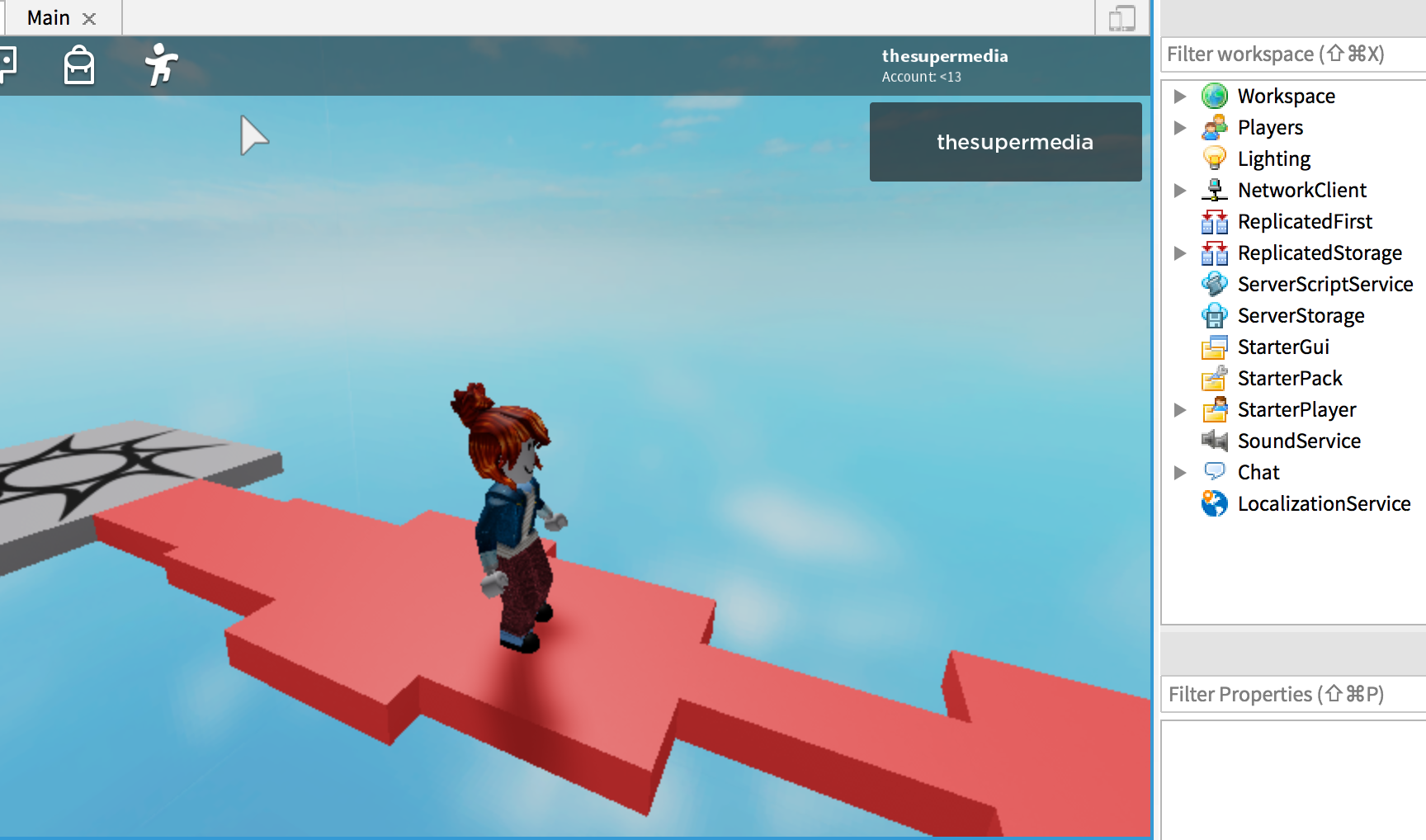Here the code to generate a path made of tiles (Part) using a single Part that sits in the ServerStorage service:

``````for count = 0, 10 do
local p = game.ServerStorage.Tile:Clone()
p.Parent = game.Workspace
p.Position = Vector3.new(0,0,-8.5 - count*5)

-- math.random(2,10) return a number between 2 and 10
p.Size = Vector3.new(math.random(2,10), 1, 5)
end
``````

The particularity of this example is the use of math.random to shuffle the size of each tile in order to get something like: